# Limits and Derivatives - Online Test

Q1.  is equal to
Explaination / Solution: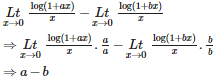Q2.  is equal to
Explaination / Solution:

Since when we put x=0, we get 0/0 form, So we have to use D'L hospital rule :

Do the differentiation of numerator and denominator partially w.r.t x , we get :

By putting x=0 directly, we get 0.

Q3. Let f (x) = x sin, x0, then the value of the function at x = 0, so that f is continuous at x = 0, is
Explaination / Solution:

Here, if we directly put x= 0, f(0) = 0 * sin (1/0) = 0.

At L.H.L, put x=0-h ,f(0-h) = = 0.

At R.H.L, put x = 0+h , , f(0+h) =  = 0.

Hence, L.H.L = f(0) = R.H.L.

f(x) is continuous at x=0.

Q4.  is equal to
Explaination / Solution: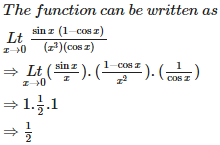Q5.  is equal to
Explaination / Solution: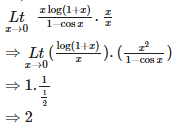Q6.   is equal to
Explaination / Solution: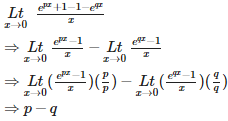Q7.  is
Explaination / Solution: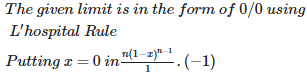Q8. is equal to
Explaination / Solution: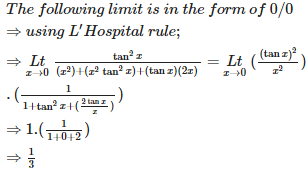Q9. Ltx0[cosx]
Explaination / Solution: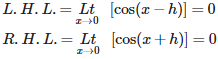Q10.  is equal to
Explaination / Solution:

Since the limit is in the form of 0/0. By applying L'hospital first time we get,

Again using L'Hospital;

Again using L'Hospital we get